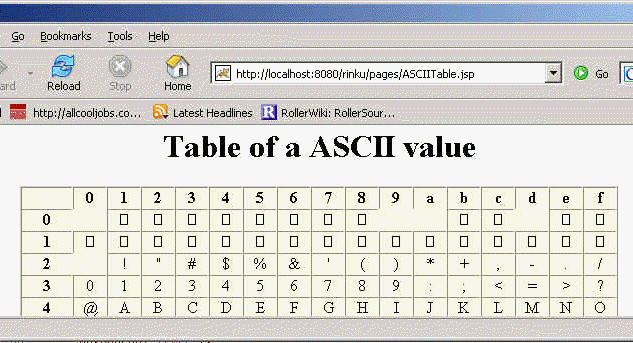Ads

Tutorials

# ASCII values table

ASCII stands for American Standard Code for Information Interchange. Computers can only understand numbers, so an ASCII code is the numerical representation of a character such as @, #, \$, and so on. ASCII was developed when non- printing characters were

ASCII stands for American Standard Code for Information Interchange. Computers can only understand numbers, so an ASCII code is the numerical representation of a character such as @, #, \$, and so on. ASCII was developed when non- printing characters were

# ASCII values table

ASCII stands for American Standard Code for Information Interchange. Computers can only understand numbers, so an ASCII code is the numerical representation of a character such as @, #, \$, and so on. ASCII was developed when non- printing characters were rarely used.

In the  example given below we have include the description of the some non- printing characters.

The code of the program is given below:

 ```<%@ page session="false" %>

Table of a ASCII value

<% StringBuffer sb = new StringBuffer(); sb.append("
"); sb.append(Integer.toHexString(col)); sb.append("
"); sb.append(Integer.toHexString(row)); sb.append(""); sb.append(c); sb.append("
"); sb.append(""); for (int col = 0; col < 16; col++) { sb.append(""); } sb.append(""); for (int row = 0; row < 16; row++) { sb.append(""); sb.append(""); for (int col = 0; col < 16; col++) { char c = (char)(row * 16 + col); sb.append(""); } sb.append(""); } out.println(sb); %> ```

Output of the Program:Download this example.

Ads

Ads